### Welcome to the Simplilearn Community

Want to join the rest of our members? Sign up right away!

# Data Science with Python | Nov 23 - Dec 11 | Ankur Aggarwal

hi

#### Lenin_7

##### Member
kNN stands for k-Nearest Neighbours. It is a supervised learning algorithm. This means that we train it under supervision. We train it using the labelled data already available to us. Given a labelled dataset consisting of observations (x,y), we would like to capture the relationship between x — the data and y — the label. More formally, we want to learn a function g : X→Y so that given an unseen observation X, g(x) can confidently predict the corresponding output Y.

As with most technological progress in the early 1900s, KNN algorithm was also born out of research done for the armed forces. Two offices of USAF School of Aviation Medicine — Fix and Hodges (1951) wrote a technical report introducing a non-parametric method for pattern classification that has since become popular as the k-nearest neighbor (kNN) algorithm.

How does it work?
Let’s say we have a dataset with two kinds of points — Label 1 and Label 2. Now given a new point in this dataset we want to figure out its label. The way it is done in kNN is by taking a majority vote of its k nearest neighbours. k can take any value between 1 and infinity but in most practical cases k is less than 30.

Blue Circles v/s Orange Triangles
Let’s say we have two groups of points — blue-circles and orange-triangles. We want to classify the Test Point = black circle with a question mark, as either a blue circle or an orange triangle.

Goal: To label the black circle.

For K = 1 we will look at the first nearest neighbor. Since we take majority vote and there is only 1 voter we assign its label to our black test point. We can see that the test point will be classified as a blue circle for k=1.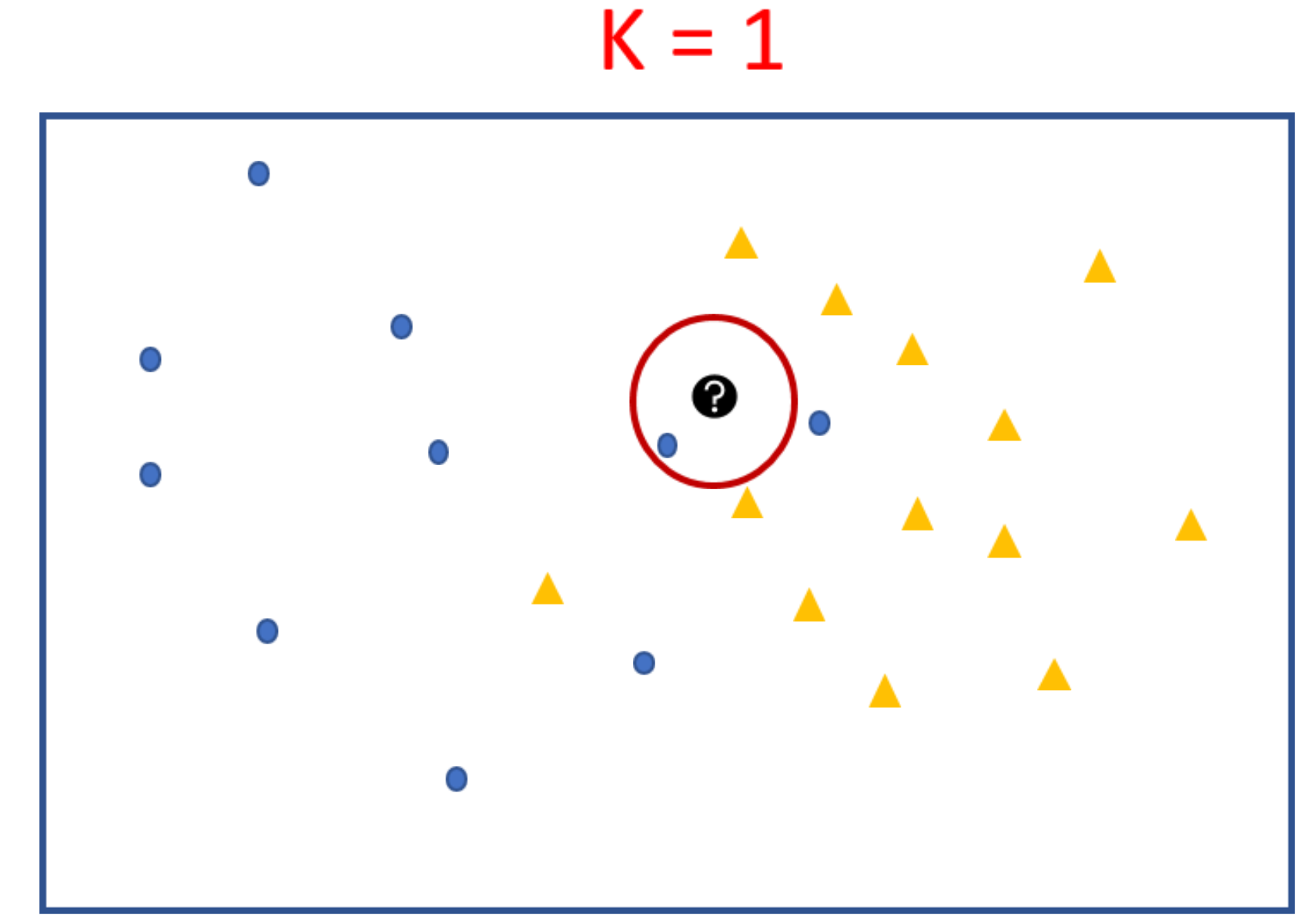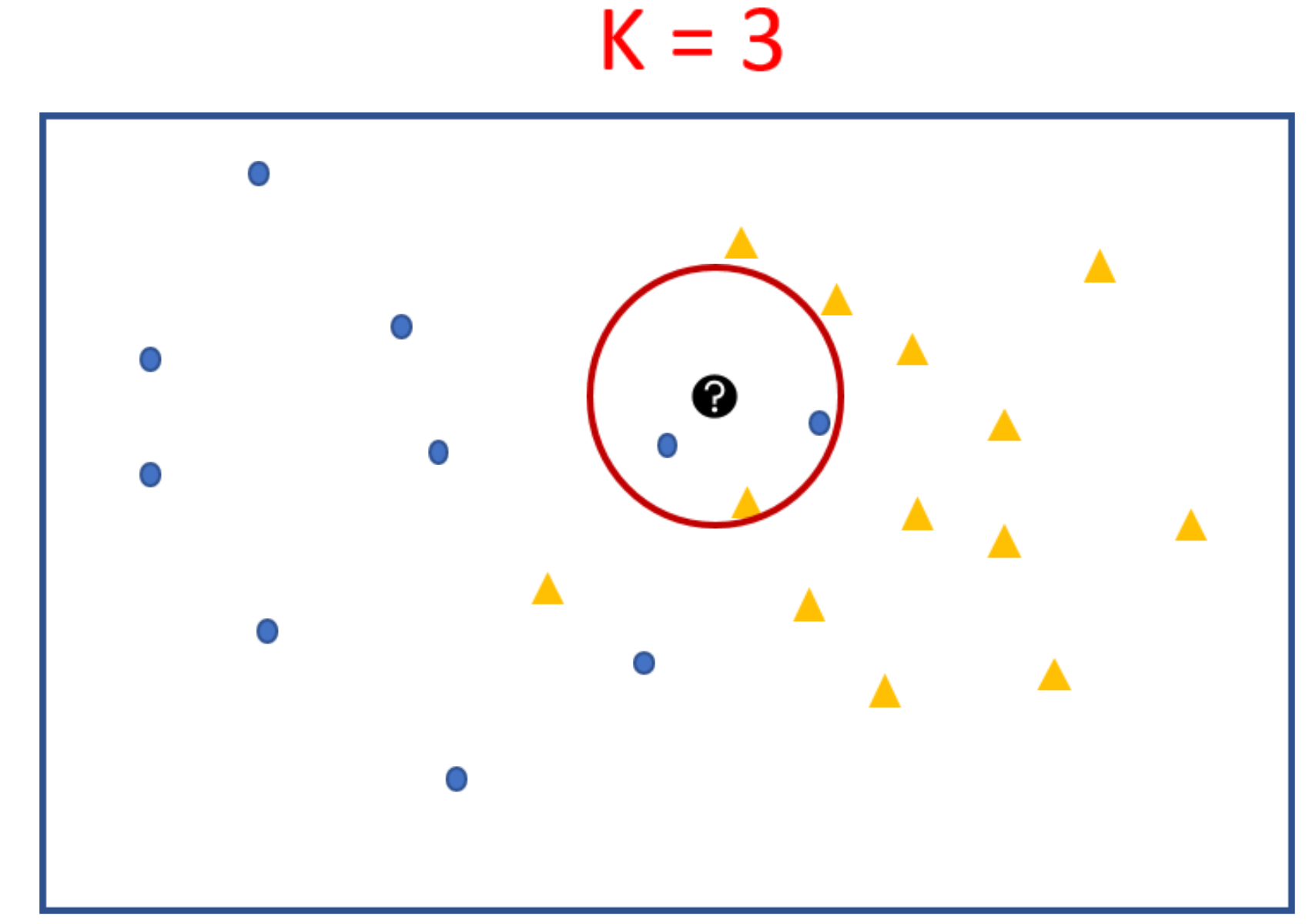Expanding our search radius to K=3 also keeps the result same, except that this time it is not an absolute majority, it’s 2 out of 3. Still with k=3 test point is predicted to have the class blue-circle →because the majority of points are blue.

Let’s see how k=5 and K =9 do. To look at the nearest neighbors we draw circle with test point at the centre and stop when 5 points fall inside the circle.

When we look at the 5 and subsequently at K = 9, the majority of the closest neighbors of our test point are orange-triangles. That indicates that the test point must be an orange triangle.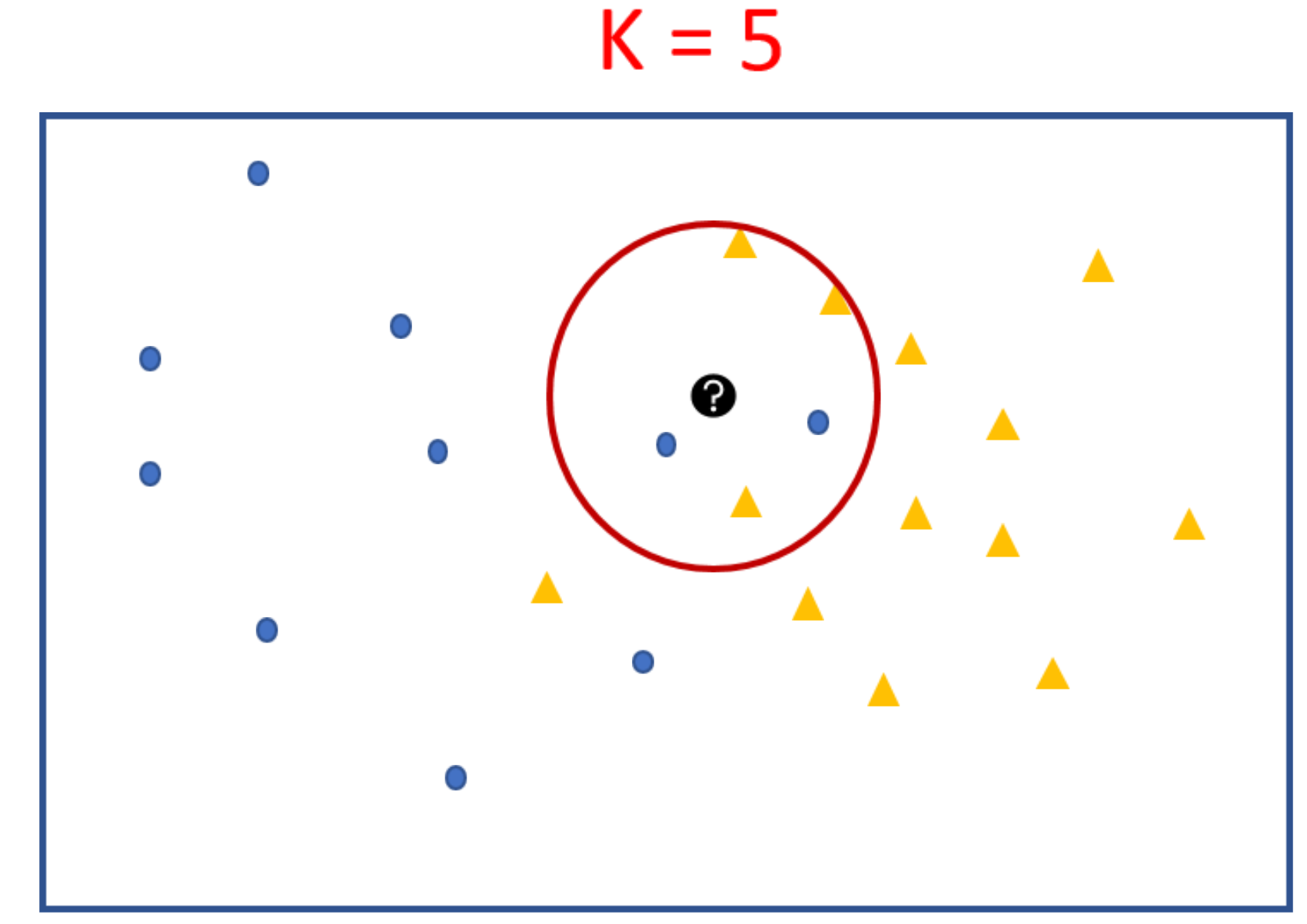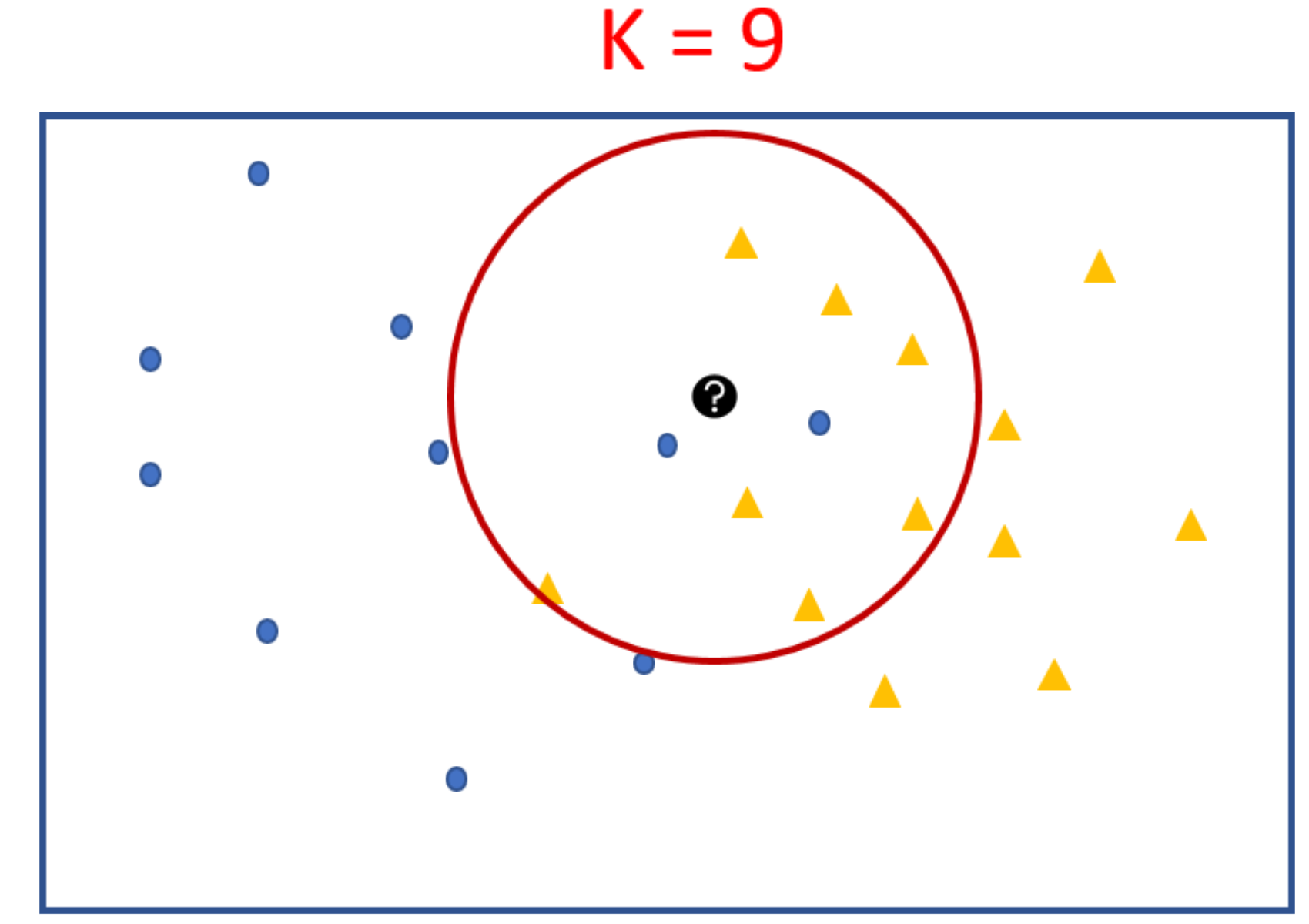Now that we have labelled this one test point we repeat the same process over all the unknown points (i.e. the test set). Once all test points are labelled using k-NN we try separating them using a decision boundary. Decision boundary shows how well the training set is separated.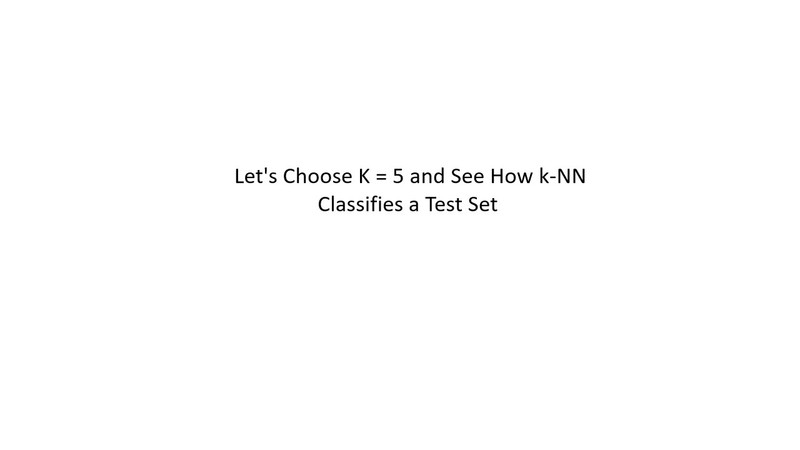That’s the gist of how k-NN happens. Let’s see it from the point of view of a machine learning engineer’s brain.
First they would choose k. We already saw above that a bigger k takes a vote of larger number of points. This means higher chances of being correct. But at what cost, you say?

Getting the k nearest neighbours means sorting through the distances. That is a costly operation. A very high processing power is needed which translates to either longer processing time or costlier processor. Higher the K costlier the whole procedure. But too low a k would result in overfitting.

A very low k will fail to generalize. A very high k is costly.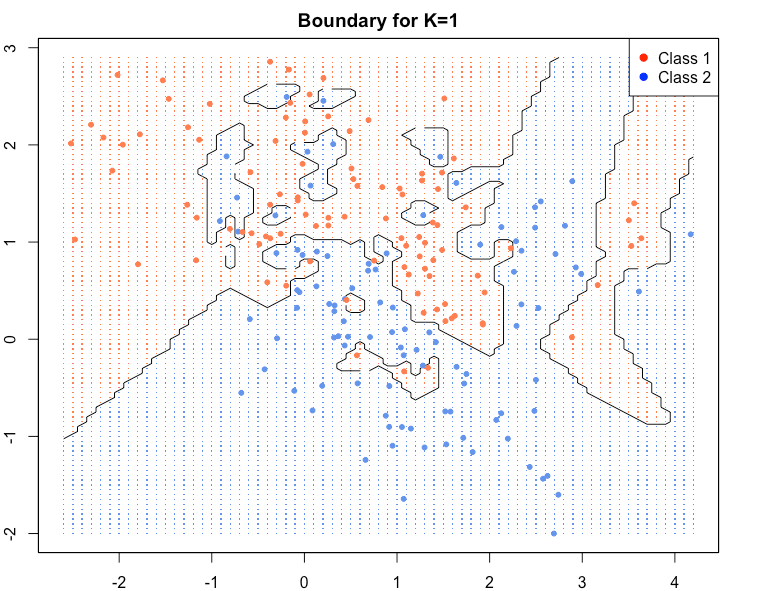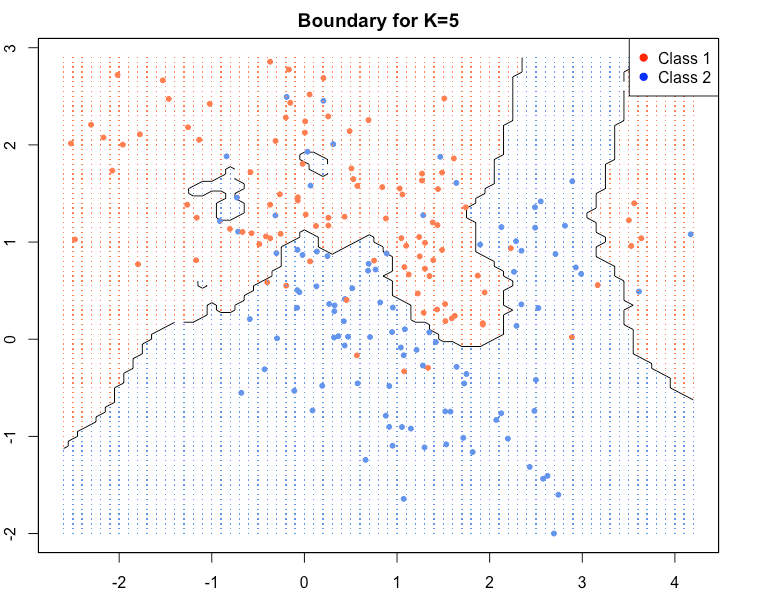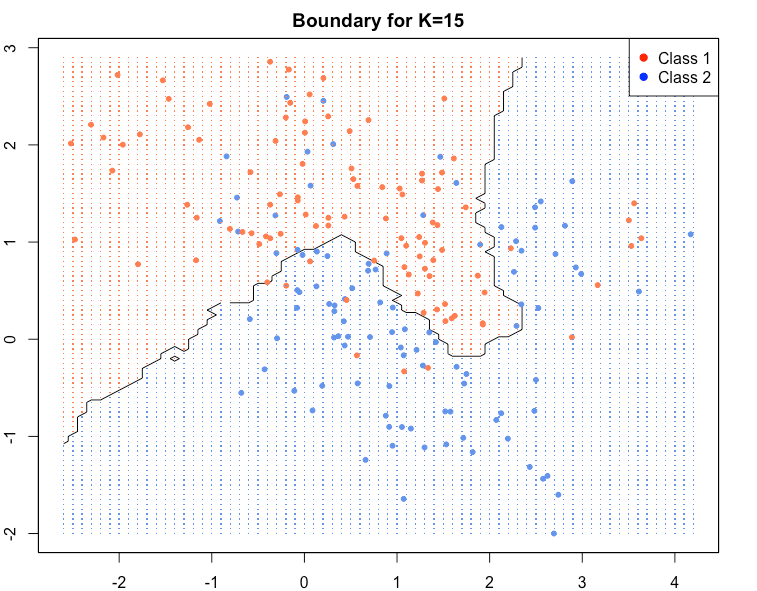As we go to higher K’s the boundaries become smooth.Blue and red regions are broadly separated. Some blue and red soldiers are left behind the enemy lines. They are collateral damage. They account for loss in training accuracy but lead to better generalisation and high test accuracy i.e. high accuracy of correct labelling for new points.

A graph of validation error when plotted against K would typically look like this. We can see that around K = 8 the error is minimum. It goes up on either side.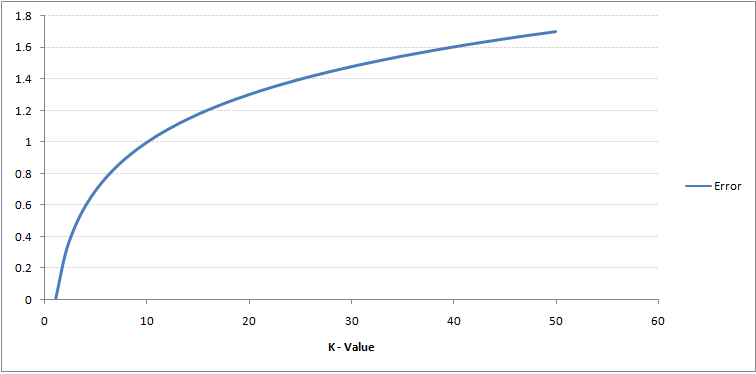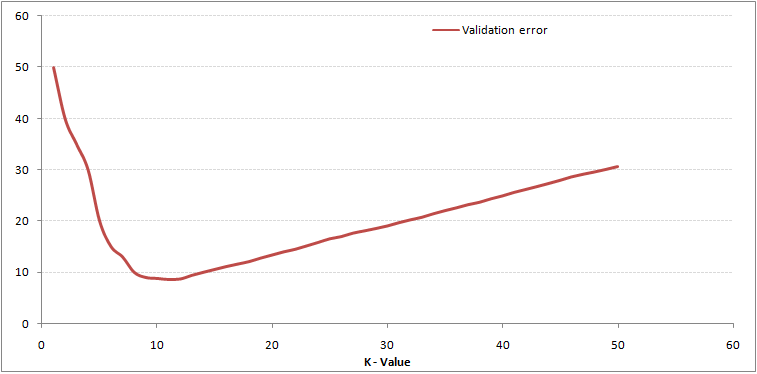Left : Training error increases with K | Right : Validation error is best for K = 8 | Source: AnalyticsVidhya
Then they would try to find distances between points. How do we decide which neighbours are near and which are not?

• Euclidean Distance — Most common distance metric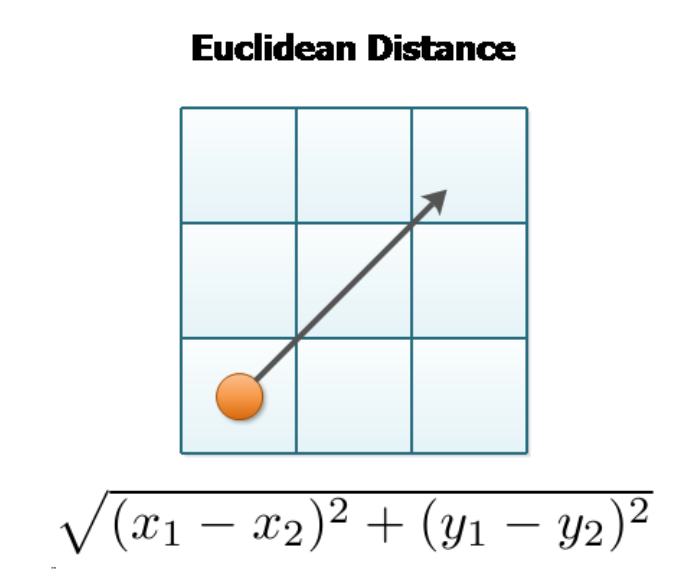Once we know how to compare points based on distance we would like to train our model. The best part about k-NN is that there is no explicit training step for it. We already know all that is to know about our dataset — its labels. In essence the training phase of the algorithm consists only of storing the feature vectors and class labels of the training samples.

K-NN is a lazy learner because it doesn’t learn a discriminative function from the training data but memorizes the training dataset instead.

An eager learner has a model fitting or training step. A lazy learner does not have a training phase.

Finally, why k-NN?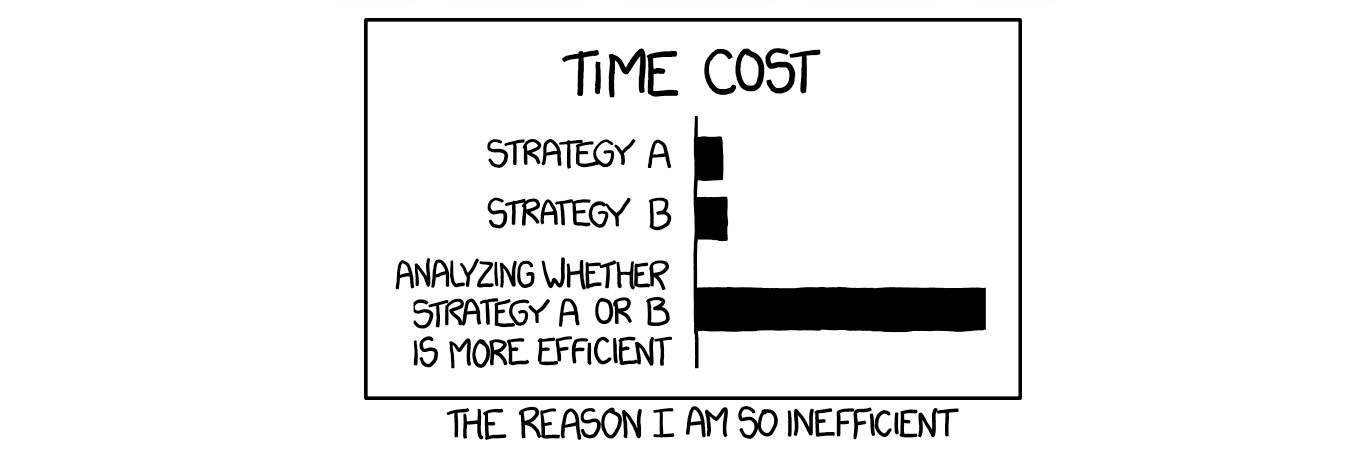• Quick to implement : Which is why it is popular as a benchmarking algorithm.
• Less training time: Faster turn around time
• Comparable accuracies: Its prediction accuracy as indicated in a lot of research papers is fairly high for a lot of applications.
k-NN is a life saver when one has to quickly deliver a solution with fairly accurate results. In most tools like MATLAB, python, R it is given as a single line command. Despite that it is very easy to implement and fun to try.

#### Lenin_7

##### Member
Hi Ankur , i would like to know ,
Are you starting any class on DS with R , in this month, So that i could register to that class . It would be helpfull

#### Sujthkumar s

##### New Member
Hi, can anyone please tell me when is the last date for submit the project and take up the Test ? as our data science with python class is extended for some more days..